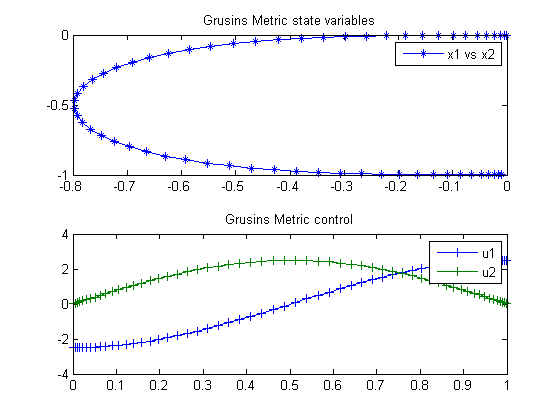47  Grusins Metric

A Short Introduction to Optimal Control, Ugo Boscain, SISSA, Italy

3.4 A Detailed Application: the Grusin’s Metric

47.1  Problem Description

Find u over t in [0; 1 ] to minimize:

J =
 1 0
u12+u22 dt

subject to:

 dx1 dt
= u1
 dx2 dt
= u2*x1
 x(t0) = [0  0 ]
 x(tf) = [−0.001  −1 ]

Reference: 

47.2  Problem setup

toms t
p = tomPhase('p', t, 0, 1, 60);
setPhase(p);

tomStates x1 x2
tomControls u1 u2

% Boundary constraints
cbnd = {initial({x1 == 0; x2 == 0})
final({x1 == -0.01; x2 == -1})};

% ODEs and path constraints
ceq = collocate({dot(x1) == u1
dot(x2) == u2.*x1});

% Objective
objective = integrate(u1.^2+u2.^2);

47.3  Solve the problem

options = struct;
options.name = 'Grusins Metric';
solution = ezsolve(objective, {cbnd, ceq}, [], options);
t  = subs(collocate(t),solution);
x1 = subs(collocate(x1),solution);
x2 = subs(collocate(x2),solution);
u1 = subs(collocate(u1),solution);
u2 = subs(collocate(u2),solution);
Problem type appears to be: qpcon
Starting numeric solver
===== * * * =================================================================== * * *
TOMLAB - Tomlab Optimization Inc. Development license  999001. Valid to 2011-02-05
=====================================================================================
Problem: ---  1: Grusins Metric                 f_k       6.233154129251588800
sum(|constr|)      0.000000060297919979
f(x_k) + sum(|constr|)      6.233154189549508400
f(x_0)      0.000000000000000000

Solver: snopt.  EXIT=0.  INFORM=1.
SNOPT 7.2-5 NLP code
Optimality conditions satisfied

FuncEv    1 ConstrEv   45 ConJacEv   45 Iter   38 MinorIter  271
CPU time: 0.593750 sec. Elapsed time: 0.610000 sec.

47.4  Plot result

subplot(2,1,1)
plot(x1,x2,'*-');
legend('x1 vs x2');
title('Grusins Metric state variables');

subplot(2,1,2)
plot(t,u1,'+-',t,u2,'+-');
legend('u1','u2');
title('Grusins Metric control');